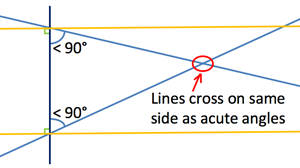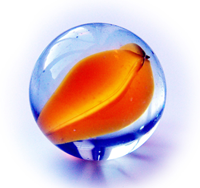## Non-Euclidean Geometry

The idea of geometry was developed by Euclid around 300 BC, when he wrote his famous book about geometry, called The Elements. In the book, he starts with 5 main postulates, or assumptions, and from these, he derives all of the other theorems of geometry. The postulates are as follows:Illustration of Fifth Postulate

1. Given two points, there is a straight line that joins them.
2. A straight line segment can be prolonged indefinitely.
3. A circle can be constructed when a point for its centre and a distance for its radius are given.
4. All right angles are equal.
5. If a straight line falling on two straight lines makes the interior angles on the same side less than two right angles, the two straight lines, if produced indefinitely, will meet on that side on which the angles are less than the two right angles. 

The fifth postulate is clearly more complicated than the other four, and, over the years, many mathematicians were upset by this fact, believing that the fifth postulate should, in some way, be possible to derive from the first four. However, in attempting to do this, they just ended up coming up with several equivalent postulates. A few are as follows:

1. Given a line and a point not on the line, it is possible to draw exactly one line through the given point parallel to the line.
2. To each triangle, there exists a similar triangle of arbitrary magnitude.
3. The sum of the angles of a triangle is equal to two right angles (180 degrees). 

Thousands of years after Euclid introduced the problem, nobody had yet come up with a proof of the fifth postulate; instead they had just come up with many postulates that were equivalent. By the mid-nineteenth century, mathematicians (Gauss, Bolyai, Lobachevsky, Reimann and Klein, to name just a few) began to explore alternative geometries, where this fifth postulate was not true.Constant Negative Curvature
Euclidean geometry assumes that there is a unique parallel line passing through a specific point; any other line will cross the original line at some point. However, you could imagine a geometry where there are many lines through a given point that never pass through the original line. This type of geometry is called hyperbolic geometry.Constant Positive Curvature
Conversely, a geometry could exist where it is impossible to draw a line that never passes through another line; this type of geometry is called elliptical. Hyperbolic geometry is a mathematical description of space of negative curvature; elliptical geometry describes space of positive curvature. 2-dimensional space of constant positive curvature is mathematically equivalent to the surface of a sphere in 3 dimensions; the representation of 2-dimensional space of constant negative curvature in 3-dimensional space could be imagined as looking something like a saddle. Because there are only three options (multiple lines that never cross the original, one line that never crosses the original, and no lines that never cross the original), there are only three basic types of geometry possible (hyperbolic, Euclidean, and elliptical, respectively); all other types are combinations of these three. The CurvedLand applet models an example of elliptical geometry; in the applet, you can see that any line you draw will eventually cross all other lines that you draw.

Curved space was simply a mathematical idea until Einstein developed his general theory of relativity in 1915 . This theory posited that, instead of being a force, gravity was the result of the curvature of space and time. Finally, the idea of curved space, or non-Euclidean geometry, had a real-world application. Although general relativity's predictions only differed from the classical model of gravity by a small amount for most observable situations, it accounted for some unexplained inconsistencies perfectly; for example, a small deviation in Mercury's orbit was not explained by the classical model of gravity, but was perfectly explained by general relativity. Another consequence of general relativity, called gravitational lensing, was observed in 1919 , shortly after the theory was proposed; this effect involves the bending of light from a distant star as it passes by a massive object, and is discussed in more detail in this article about gravitational lensing published by NASA, and in this video on YouTube, produced as part of the Cosmic Cinema series by the Max Planck Institute of Astrophysics. These observations support the validity of general relativity, and the actual existence of curved space-time, giving tangible reasons why we should try to better understand non-Euclidean geometry.